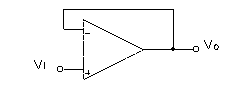EE 321 Lab

Lab 2: OPERATIONAL AMPLIFIERS PART I -- FREQUENCY CHARACTERISTICS

Doing the prelab before coming to lab will get you out on time. Waiting until your lab session WILL KEEP YOU IN THE LAB WELL PAST DINNER.

The purpose of this lab is to examine the frequency response of the operational amplifier. Its finite frequency response will be investigated in a simple inverting operational amplifier circuit. The operational amplifier and the inverting amplifier will be found to behave like a single time constant low pass filter.

Inverting Amplifiers

1. Construct an inverting amplifier (designed as part of the pre lab) having an input resistance of 1 kohms and a gain of about 5. Use the 741 op-amp with +-15 Volt supply voltages. Employ good breadboard technique by using the long bus lines on your protoboard for each of the supply voltages and ground.

2. Apply a 1 kHz sine wave to the input. Display both the input and output on the scope. a) Measure the gain and compare with the theoretical gain. b) Confirm that the op-amp inverts the input by displaying both input and output on the scope (sketch waveforms). c) Vary the d.c. level of the input sine wave (offset) to confirm that the amplifier responds to d.c. inputs. d) Increase the input amplitude to determine the d.c. output voltage levels at which the output `saturates'.

These test can be quickly done to make sure your amplifier is working correctly.

Slew Rate

3. Change the input to a square wave. At 100 Hz adjust the output to be 20 V p-p with no saturation or d.c. level. Now increase the signal frequency to about 100 kHz. What has happened to the output? The output of the op amp can not change faster than a certain rate, called the `slew rate'. Measure the slew rate of this 741 op amp (the slope in Volts/us)? Compare with the value given in the op amp specs.

4. Change the input back to a sin wave. Decrease the frequency until the 20 V p-p sinusoidal output can be obtained without distortion. Find the frequency where the distortion just begins (don't try to get too close). This is related to the slew rate of the op amp as measured in the preceding part. SR=wM Vomax, where Vomax is the amplitude of the output voltage. (p. 100 of Sedra and Smith). Check this relationship.

5. Repeat either one of these slew rate measurements for the 411 op amp. Compare this result to the data sheet.

Open Loop Gain - The gain of the op amp itself.

6. The finite gain of an op amp may be determined by measuring the small voltage at the (-) input of the op-amp. Re-construct the 741 inverting amplifier and increase the gain to 10. Set the output voltage to be 20 V p-p at a frequency of 1 kHz. Sketch the circuit. Measure the voltage at the inverting input of the op-amp with the X1 probe. What op amp gain does this imply (it should be near 1000)? Repeat at frequencies of 10 kHz and 100 kHz. If necessary decrease the output if slew rate causes distortion. How does the op-amp gain appear to vary with frequency?

7. At 10 kHz note the phase shift between Vout and V-. What is its approximate value, and why is it present?

Frequency Response of the Inverting Amplifer with Gain 10

8. Use the same circuit as 6) and 7). To make sure there won't be any distortion during this measurement, set the output voltage to be near 0.1 V p-p at a frequency of 1 MHz. If necessary, decrease the signal amplitude, so that the slew rate does not cause distortion. Measure the frequency response or cutoff frequency f0 of the circuit by adjusting the frequency until the gain is .707 of its low frequency value (measure the output at 100 Hz, then increase the frequency until the output drops by a factor of 0.707).

Why is the cutoff frequency also called the `half power' frequency or the 3dB frequency?

Find ft using Eq (2.27) in Sedra and Smith (f0 is the same as f3dB). Compare your ft to that in the data sheet.9. Determine the unity gain bandwidth fT of the 741 op amp by connecting it as a unity gain follower and measuring the 3 dB (half power) frequency. Use the X10 probe for measurements above 1 MHz. Keep the output amplitude small to ensure that the slew rate is not affecting the signal amplitude. Compare with specs.

10. Find the unity gain bandwidth fT for the 411.

Comparison of op amps.

11. From the data obtained so far, which type of op amp, the 741 or 411, is better and why? Which is closer to an ideal op-amp? Which has a more informative spec sheet?

Extra credit

12. Given the measured value of fT, what 3 dB frequency would you expect for the 411 connected to have a non-inverting gain of 10 and 100? Test one of these predictions by actual measurement.